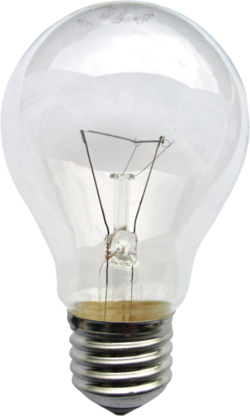# Electric powerFigure 1. A typical incandescent light bulb. The filament is the thin wire stretched between the vertical contact wires and held up by two other support wires. The electric power is given by the electric current times the voltage drop across the light bulb.

Electric power is a transfer of energy over time (just like un-prefixed power), however, it specifically refers to energy transfer in the form of electricity, sending electric current through conductors.

Power = Current x Voltage

Power tools, vacuum cleaners, and wall chargers all have an "amps" rating on them. The amperage (A) of a normally operating industrial circular saw is 15 A. With this amperage rating, it is possible to determine the total power output of the tool, simply by multiplying the amperage by the voltage of the current it is drawing. For example, running a 15 A circular saw off of a 120 V circuit would lead to a power draw of 1800 watts (W).

## Power

Electric power is how fast an electrical component, electric circuit or system uses energy.

• Power units are the Watt (W), 1 watt is equivalent to 1 Joule/second (J/s).

The following relationship emerges between power, current and applied voltage:

Where is the power, is the circuit current and is the voltage change across the component. With the help of Ohm's law the power equation can be manipulated to be put in terms of different variables as described in the table below.

In terms of Formula
circuit current and applied voltage
circuit current and circuit resistance
applied voltage and resistance

The power rating is crucial in electrical applications especially to transducers.

### Power rating

The power rating is the maximum rate at which an electronic device (for example a transducer) can convert energy into some other form, similar to ampacity for electric current. Any device which is capable of converting one form of energy to another is referred to as a transducer. For example, resistors have the ability to absorb energy and transform it into heat. The rate at which it absorbs energy must be equal to the rate at which it dissipates heat. If the rate at which the resistor absorbs heat is exceeded the resistor will melt, and be destroyed. The power rating for the resistor thus describes its ability to dissipate heat.

### Power efficiency

The power efficiency of a device is a measure of how well that device converts electricity into work. Electric motors have extremely high power efficiency, usually over 90%, and often exceeding 96%. This is in stark contrast to internal combustion engines, which have an efficiency of between 25% and 35%. This discrepancy in efficiency is why electric vehicles achieve such high MPGe ratings. This is also why electricity is considered to be higher quality energy.

Power efficiency cannot exceed 100% because energy cannot be created or destroyed as stated in the First law of thermodynamics.

## For Further Reading

For further information please see the related pages below:

## Authors and Editors

Bethel Afework, Jordan Hanania, James Jenden, Kailyn Stenhouse, Jason Donev
Last updated: May 18, 2018
Get Citation